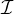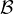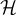#### 2.1 Basic Nomenclature

Our computational model implies that the data allocation on the parallel distributed memory machine is guided by the structure of the physical model, and specifically by the discretization mesh of the PDE.

Each point of the discretization mesh will have (at least) one associated equation/variable, and therefore one index. We say that point i depends on point j if the equation for a variable associated with i contains a term in j, or equivalently if aij0. After the partition of the discretization mesh into sub-domains assigned to the parallel processes, we classify the points of a given sub-domain as following.

Internal.
An internal point of a given domain depends only on points of the same domain. If all points of a domain are assigned to one process, then a computational step (e.g., a matrix-vector product) of the equations associated with the internal points requires no data items from other domains and no communications.
Boundary.
A point of a given domain is a boundary point if it depends on points belonging to other domains.
Halo.
A halo point for a given domain is a point belonging to another domain such that there is a boundary point which depends on it. Whenever performing a computational step, such as a matrix-vector product, the values associated with halo points are requested from other domains. A boundary point of a given domain is usually a halo point for some other domain2 ; therefore the cardinality of the boundary points set denotes the amount of data sent to other domains.
Overlap.
An overlap point is a boundary point assigned to multiple domains. Any operation that involves an overlap point has to be replicated for each assignment.

Overlap points do not usually exist in the basic data distributions; however they are a feature of Domain Decomposition Schwarz preconditioners which are the subject of related research work .

We denote the sets of internal, boundary and halo points for a given subdomain by,and. Each subdomain is assigned to one process; each process usually owns one subdomain, although the user may choose to assign more than one subdomain to a process. If each process i owns one subdomain, the number of rows in the local sparse matrix is |i| + |i|, and the number of local columns (i.e. those for which there exists at least one non-zero entry in the local rows) is |i| + |i| + |i|.Figure 2: Point classfication.

This classification of mesh points guides the naming scheme that we adopted in the library internals and in the data structures. We explicitly note that “Halo” points are also often called “ghost” points in the literature.